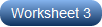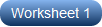# Decimal and Binary Conversion Worksheets

Learn the language of the computers with our printable decimal and binary conversion worksheets. Emphasize the difference between base-2 and base-10 systems, and get high school students to practice the simple steps involved in converting the numbers from binary to decimal and decimal to binary in these pdfs. Remember, each digit from the right to left in a binary number increases by a factor of 2, whereas each digit in a decimal, increases by a factor of 10. Our free decimal and binary conversion worksheet is a great place to start your practice.

Converting Decimal to Binary

Switching from base-10 to base-2 is easy! Divide the number by 2 repeatedly until the quotient is 0, write down the remainders that are a series of bits: 1s and 0s. Arrange the remainders from the last to the first, write them with a base 2, and you are done!Converting Binary to Decimal

Translate the binary numbers to decimal numbers in our pdf worksheets. The decimal number is equal to the sum of binary digits (bn) times their power of 2 (2n): b0x20 + b1x21 +b2x22 and so on. Begin with the right most binary number, and decode!Converting between Decimal and Binary | MCQ

High school students are in for a tremendous challenge presented in these printable converting between decimal and binary worksheets. Spot the decimal equivalent of a binary number or the binary equivalent of a decimal as directed.